# STAT3009 Project 1¶

Name: Liu Mei Suet, Michelle

Sid: 1155142156

## Section 0: Introduction¶

This project aims to build a recommendation mechanism based on Matrix Factorization. The dataset contains 3 columns (i.e. User_ID, Item_ID, and rating) over 16000 rows (i.e. around 800 items and 600 users). For the result, the rating of each user-item pair in test dataset will be predicted and the top 5 preferred items for the user with ID 18 (156-th record in test dataset) will be provided.

## Section 1: Exploratory data analysis (EDA)¶

To begin with, I would like to perform some preliminary analyses to get an overview of the pattern and characteristics of data, which would benefit the model development.

The dataset contains below columns:

• User ID

• Item ID

• Rating (0 to 10)

Basic descriptions of the dataset:

• Data type: integer

• Total number of users: 600

• Total number of items: 799
• User with id 9 rated the most items
• Item with id 786 is rated the most

Let’s visualize the data related to user 9 and item 786 respectively to see if there is any noticeable pattern. The following are the histograms of their ratings.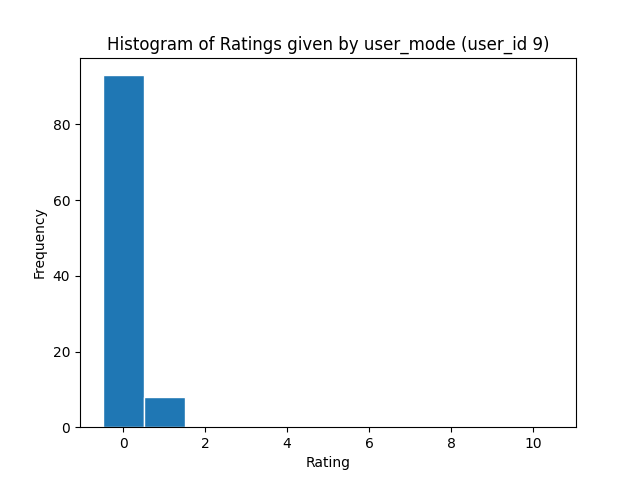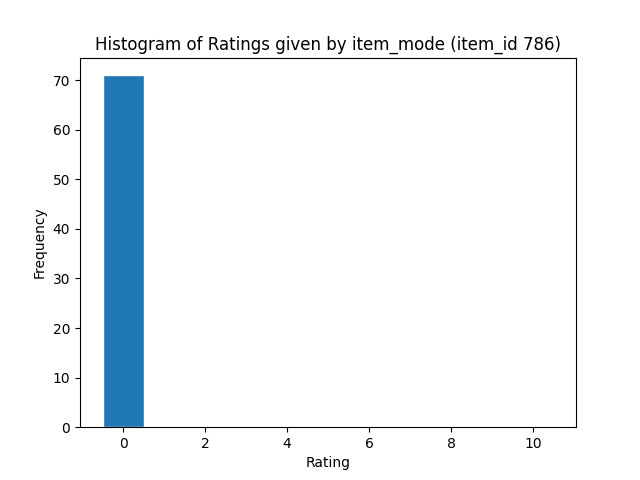Insight:

It is noticeable that user 9 (user_mode) rated most of the items with 0 and a few items with 1. More interestingly, all ratings of item 786 (item_mode) are 0. To investigate if the other users and items exhibit this extreme pattern, I would like to check the variance of ratings of items and ratings given by users in the training set.

### Visualization 2: Variance of ratings of items and ratings given by users¶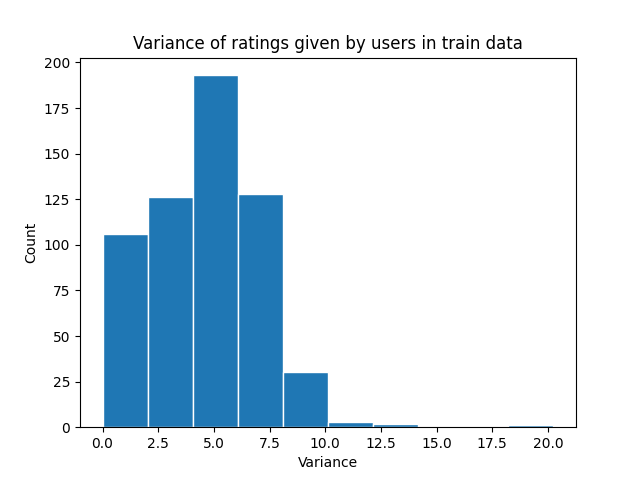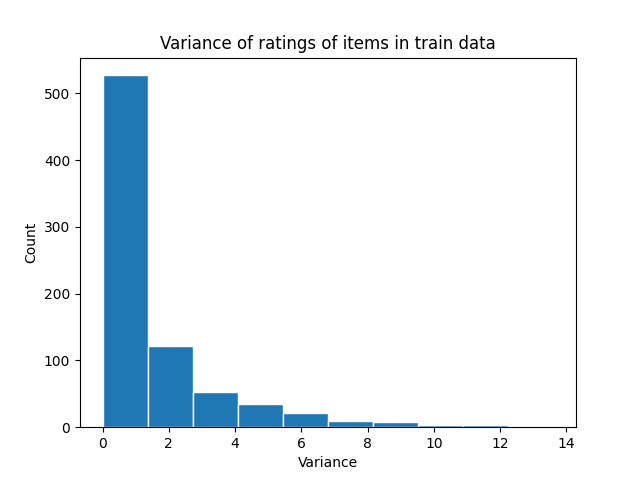Insight:

Through the histograms, we can observe that

1. Many users have 0 - 5 variance with their ratings, which implies that quite a lot of users gave the same or similar ratings to all the items they watched
1. Many items have 0 variance with their ratings and almost all of them have a variance less than 5, which implies that many items received the same ratings and more importantly, the deviation from the mean is less than $\sqrt{5}$ for most of the items.

Thus, user mean and item mean should be useful in making predictions.

### Visualization 3: Distribution of Ratings¶

Apart from the variance, I would like to have a look at the distribution of ratings to see whether users have any tendency to rate.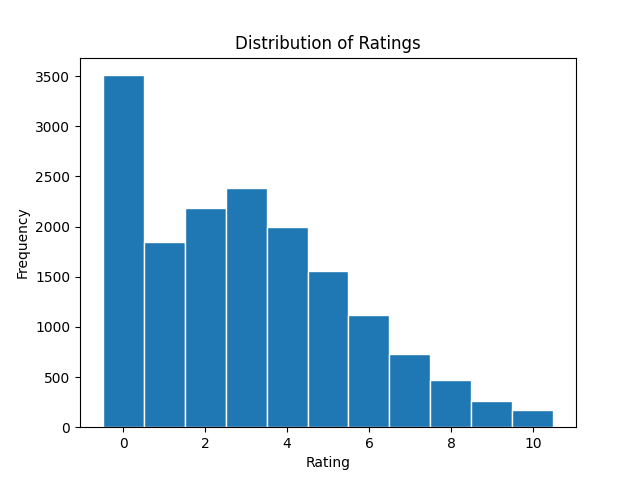Insight:

In this plot, we can see that

• The most common rating that users give is 0 and the second most common rating is 3
• Only a few people rated 8 or above

Based on these, we can conclude that users tend to give lower ratings, especially 0.

### Visualization 4: Popularity of Items and Activeness of Users¶

The popularity of item and the activeness of user may also be taken into consideration as they provide useful information on the dataset. Usually, a high-quality item may easily attract many users so that the item will receive more ratings and these ratings are often quite high. On the other hand, as an active user has tasted many items before, a new item is difficult to attract the user’s attention, and thus he/she may tend to give a rating falling into a small range (i.e. between rate 3-5) instead of a wider range (i.e. between rate 1 to 10).

To analyze, I plot the average rating of each item and each user against the number of ratings received by each item and ratings given by each user.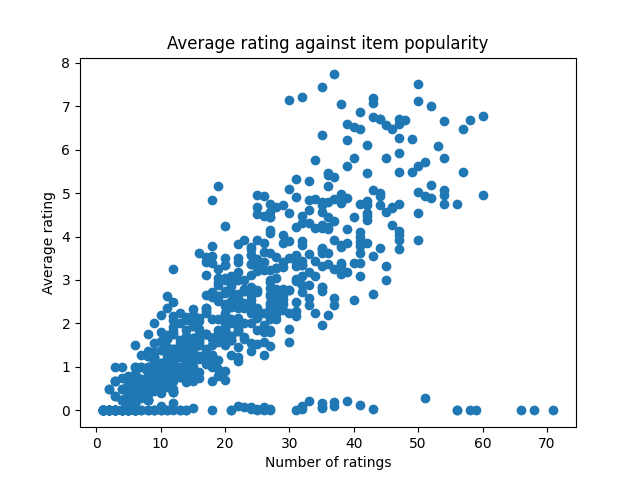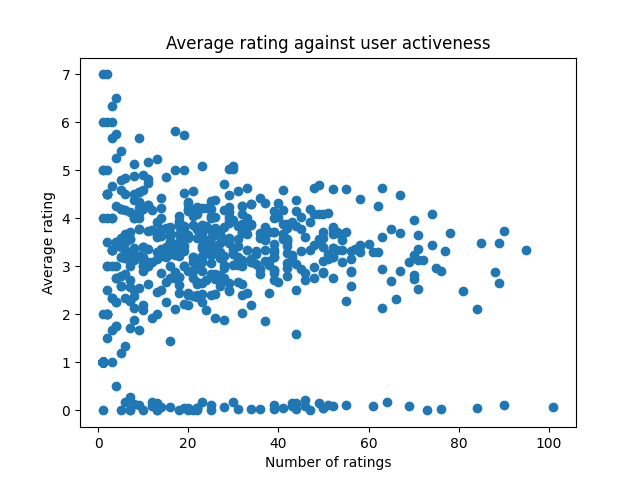Insight:

The item popularity scatter plot shows a pattern that the average rating increases when the number of ratings of an item increases (i.e. when an item becomes more popular). On the other hand, the user activeness scatter plot shows a pattern that when the number of ratings given by the user increases, the average rating seems to be converging to a certain value (i.e. rate 3 - 5).

More importantly, just as what we have observed in visualization 3, there are quite a number of users who give a rate 0 to all the items and plenty of items received all 0 ratings. Thus, special treatment may be taken to due with these patterns during model development.

### Summary of Exploratory data analysis (EDA)¶

Visualizations 1 and 4 suggest the following findings which may be useful in developing the model:

• User mean and item mean can be used to explain some information given by the train dataset
• Average rating is positively correlated with item popularity
• Average rating may converge to a certain value when the number of ratings given by users increase
• Some users gave a 0 rating to all items they rated and some items received 0 rating from all rated users, which implies to apply special treatment for some set of user_id and item_id.

# Section 2: Model development¶

Two main models to use in this section are:

• SVD
• SVD++

As mentioned in Section 1, the item mean and user mean would be useful to explain the data, residual based method is used to incorporate item and user mean in our SVD or SVD++ model.

Remark: In this project, the Package Surprise (i.e. a “scikit-based Python” libraries to develop recommender systems) is used to implement SVD and SVD++ model.

#### Model 1: SVD Model¶

To begin with, let’s consider the SVD algorithm below.

The predicted rating is given as: $$\widehat{r}_{ui} = \mu + {b}_u + {b}_i + \mathbf{p}_u^T \mathbf{q}_i,$$

where $({b}_u, \mathbf{p}_u); (u=1, \cdots, n)$ and $({b}_i, \mathbf{q}_i); (i=1,\cdots,m); \mathbf{p}_u$ and $\mathbf{q}_i$ are vectors of length ${k}$.

If user ${u}$ is unknown, then the bias ${b}_u$ and the factors ${p}_u$ are assumed to be zero. The same applies for item $i$ with ${b}_i$ and ${q}_i$.

To estimate all the unknown, we minimize the following regularized squared error: $$\sum_{(u,i) \in \Omega} ( r_{ui} - \mu - {b}_u - {b}_i - \mathbf{p}_u^T \mathbf{q}_i )^2 + \lambda \big( {b}_u^2 + {b}_i^2 + \|\mathbf{p}_u\|_2^2 + \|\mathbf{q}_i\|_2^2 \big).$$

The minimization is performed by a very straightforward stochastic gradient descent: \begin{split}b_u &\leftarrow b_u &+ \gamma (e_{ui} - \lambda b_u)\\ b_i &\leftarrow b_i & + \gamma (e_{ui} - \lambda b_i)\\ p_u &\leftarrow p_u & + \gamma (e_{ui} \cdot q_i - \lambda p_u)\\ q_i &\leftarrow q_i & + \gamma (e_{ui} \cdot p_u - \lambda q_i)\end{split}

where $e_{ui} = r_{ui} - \hat{r}_{ui}$.

The hyperparameters of these models includes:

1. the length of $\mathbf{p}_u$ and $\mathbf{q}_i$, ${k}$ ($n\_factors$)

2. the learning rate $\gamma$ for ${b}_u$ ($lr\_bu$), for ${b}_i$ ($lr\_bi$), for ${p}_u$ ($lr\_pu$), and for ${q}_i$ ($lr\_qi$)

3. the regularization term for $\lambda$ ${b}_u$ ($reg\_bu$), for ${b}_i$ ($reg\_bi$), for ${p}_u$ ($reg\_pu$), and for ${q}_i$ ($reg\_qi$)

4. the number of iteration of the SGD procedure ($n\_epochs$)

##### Model 1.1: SVD Model¶

Hyperparameters tuning -- Grid Search

To find out the optimal hyperparameters for the SVD model, I use the GridSearchCV() in the Surprise Package to find out the best parameters based on the cross-validation method.

The parameter tuning begins with a wide and sparse grid and the grid will narrow down to the local region of parameters. If a parameter stops at the boundary of the grid after an iteration, I will enlarge the grid of that parameter to explore more possibilities. The tuning will end when the best set of parameters (i.e. generate an acceptable low 'valid rmse' value) is found.

First, the parameter tuning begins with the following grid.

param_grid = {
"n_factors": [3, 5, 10, 40, 70, 100],
"n_epochs": ,
"lr_all": [0.0001, 0.001, 0.005, 0.01, 0.1],
"reg_all": [0.0001, 0.001, 0.1, 0.5, 1, 2],
"reg_bu": ,
"reg_bi": ,
}

After plenty of tuning, I finalize the parameter set as follows.

Final parameter set, valid rmse, and score in Leaderboard

param_grid = {
"n_factors": ,
"n_epochs": ,
"lr_all": [0.005],
"reg_all": [0.1],
"reg_bu": ,
"reg_bi": ,
}

The valid rmse for this attempt is 0.6839 and the score in Public Leaderboard is 0.66779.

Issue of GridSearchCV

As the SVD model involves too many hyperparameters, it would be difficult and would take too much time to tune the hyperparameters to optimal with GridSearch manually.

Improvement

Because of this issue, an automated method becomes necessary in these optimization steps. Thus, I would like to improve the hyperparameter tuning step by using a Python library called auto-surprise to find an optimal parameter set.

##### Model 1.2: SVD Model¶

Hyperparameters tuning -- Auto Surprise

Auto-Surprise is a python library that automates algorithm selection and hyperparameter optimization in a highly parallelized manner. It is built on the popular library Surprise for recommender algorithms and Hyperopt for hyperparameter tuning.

Currently, three algorithms are implemented in hyperopt:

• Tree of Parzen Estimators (TPE)
• Random Search

I have tried all three algorithms to do the automated hyperparameter optimization. The valid rmse values are as follows.

Tree of Parzen Estimators (TPE) Adaptive PTE Random Search
Max_evals = 100 0.7033 0.6740 0.7296

Remark: The maximum number of evaluations each algorithm gets for hyper parameter optimization max_evals is seted as 100.

Final parameter set, valid rmse, and score in Leaderboard

From the above table, only the parameter set generated by APTE algorithm gives a smaller valid rmse than that of Model 1.1, I use this parameter set as the final set for Model 1.2.

best_params = {'lr_bi': 0.03566094677967572,
'lr_bu': 0.028341147137220187,
'lr_pu': 0.049421104542630157,
'lr_qi': 0.024081217368581088,
'n_epochs': 106,
'n_factors': 1,
'reg_bi': 0.009582782844206644,
'reg_bu': 0.00011494409326701549,
'reg_pu': 0.02323855631873504,
'reg_qi': 0.047742294740985826
}

The valid rmse for this attempt is 0.6740 and the score in Public Leaderboard is 0.67215.

Issue of this model

The score on Leaderboard is lower than the result of Model 1.1. I guess the reason is that max_evals = 100 setting is not large enough to search the optimal parameter set.

Improvement

Because of the above issue, I would like to enlarge the max_evals in the following model. Also, from Model 1.2, the performance of Random Search (i.e. rmse = 0.7296) is much worse than that of TPE (i.e. rmse = 0.7033 ) and APTE (i.e. rmse = 0.6740). Therefore, I decided to only use TPE and APTE to automate hyperparameter optimization in the next model.

##### Model 1.3: SVD Model¶

Hyperparameters tuning -- Auto Surprise (Update the setting of max_evals)

I would like to first enlarge the max_evals from 100 to 500 and see whether valid rmse will decrease.

The valid rmse values for max_evals = 500 are as follows.

Tree of Parzen Estimators (TPE) Adaptive PTE
Max_evals = 100 0.7033 0.6740
Max_evals = 500 0.6639 0.6629

In this table, we can see that the valid rmse values decrease when the maximum evaluation increases. To be precise, rmse of TPE and APTE algorithms decreased to 0.6639 and 0.6629 respectively, which is a good improvement compared to the result of Model 1.1 and Model 1.2.

Final parameter set, valid rmse, and score in Leaderboard

The following are the final parameter sets for TPE and APTE algorithms.

# Parameter set for TPE
best_params = {'lr_bi': 0.024300743378841992,
'lr_bu': 0.0011815948581443635,
'lr_pu':0.012482710076706212,
'lr_qi': 0.00823562527513479,
'n_epochs': 143,
'n_factors': 1,
'reg_bi':0.0010038894084862182,
'reg_bu': 0.0003472980363023683,
'reg_pu': 0.0004453249677069114,
'reg_qi':0.0003207232594835713
}

# Parameter set for ATPE
best_params =  {'lr_bi': 0.019090101711133622,
'lr_bu': 0.015683258397985133,
'lr_pu': 0.025778789373718986,
'lr_qi': 0.002495939257442814,
'n_epochs': 198,
'n_factors': 1,
'reg_bi': 0.00022237810533239877,
'reg_bu': 0.0003240391997232606,
'reg_pu': 0.005122792017321656,
'reg_qi': 0.09904016788429931
}

The table below shows the valid terms and Leaderboard score for TPE and APTE.

Tree of Parzen Estimators (TPE) Adaptive PTE
Valid rmse 0.6639 0.6629

Apart from a decrease in the rmse value, we can also observe that there is a big improvement in terms of the leaderboard score, compared to 0.66779 in Model 1.1 and 0.67215 in Model 1.2.

\ Improvement

The decreasing trend in rmse implies that a larger max_evals setting can improve the performance of automated hyperparameter optimization to search for a better parameter set. Therefore, I would like to further enlarge the max_evals setting in the next model.

On the other hand, I observed that APTE always generates a parameter set with lower rmse than TPE. To lower the searching time, I decided to use APTE only to do the automated hyperparameter optimization in the next model.

##### Model 1.4: SVD Model¶

Hyperparameters tuning -- Auto Surprise (Further update the setting of max_evals)

I would like to enlarge the max_evals from 500 to 700, 1000, 1300, and 1500 and see whether valid rmse will decrease further.

The valid rmse values for different max_evals settings are as follows.

Max_evals = 100 0.6740
Max_evals = 500 0.6629
Max_evals = 700 0.6618
Max_evals = 1000 0.6583
Max_evals = 1300 0.6562
Max_evals = 1500 0.6569

In this table, we can see that the valid rmse values decrease sharply when the maximum evaluation further increases and rmse reaches the optimal, 0.6562, when max_evals = 1300.

Final parameter set, valid rmse, and score in Leaderboard

As mentioned above, valid rmse is the smallest when max_evals = 1300. I would like to use the corresponding parameter set as the final set, as shown below.

best_params = {'lr_bi': 0.023726067831278404,
'lr_bu': 0.00019672517575026568,
'lr_pu': 0.004848241381931481,
'lr_qi': 0.003689737817264781,
'n_epochs': 162,
'n_factors': 1,
'reg_bi': 0.00033922470306219375,
'reg_bu': 0.0789915548366538,
'reg_pu': 0.008997208546887707,
'reg_qi': 0.004304762657110084
}

The valid rmse is 0.6562 and the score in Public Leaderboard is 0.64861.

The table below compares the valid rmse and Leaderboard score for Model 1.1 to Model 1.4.

Model 1.1 (GridSearch) 0.6839 0.66779
Model 1.2 (Auto-Surprise, APTE, max_evals = 100) 0.6740 0.67215
Model 1.3 (Auto-Surprise, APTE, max_evals = 500) 0.6629 0.65717
Model 1.4 (Auto-Surprise, APTE, max_evals = 1000) 0.6562 0.64861

From the table, we can see that Model 1.4 has a very big improvement in terms of performance in the Leaderboard.

Issue of this model

Although we have found out a high-quality parameter set in this model, Model 1.4 is not the best model to explain the dataset.

Recall part of the summary of EDA:

• User mean and item mean can be used to explain some information given by the train set
• Average rating is positively correlated with item popularity

However, Model 1.4 doesn't takes into account the item popularity and doesn't incorporate item and user mean.

Improvement

Therefore, I would like to use another model which takes into account the item popularity. That is SVD++ Model.

#### Model 2: SVD++ Model¶

Consider a more complex model, SVD++, which takes into account the item popularity, The predicted rating is given as:

$$\widehat{r}_{ui} = \mu + b_u + b_i + \mathbf{q}^T_i \big(\mathbf{p}_u+\frac{1}{\sqrt{|\mathcal{I}_u|}}\sum_{j\in\mathcal{I}_u}\mathbf{w}_j\big),$$

where $(b_u, \mathbf{p}_u); (u=1, \cdots, n)$ and $(b_i, \mathbf{q}_i); (i=1,\cdots,m); \mathbf{p}_u$ and $\mathbf{q}_i$ are vectors of length ${k}$; $\mathbf{w}_j$ terms are a new set of item factors that capture implicit ratings. (An implicit rating describes the fact that a user ${u}$ rated an item ${j}$, regardless of the rating value.)

Just as SVD model, if user ${u}$ is unknown, then the bias ${b}_u$ and the factors ${p}_u$ are assumed to be zero. The same applies for item $i$ with ${b}_i$ and ${q}_i$.

To estimate all the unknown, we minimize the following regularized squared error: $$\sum_{(u,i) \in \Omega} \left( r_{ui} - \mu - b_u - b_i - \mathbf{q}^T_i(\mathbf{p}_u+\frac{1}{\sqrt{|\mathcal{I}_u|}}\sum_{j\in\mathcal{I}_u}\mathbf{w}_j) \right)^2 + \lambda \big( {b}_u^2 + {b}_i^2 + \|\mathbf{p}_u\|_2^2 + \|\mathbf{q}_i\|_2^2 + \sum_{j \in I_u} \|\mathbf{w}_j\|^2\big). \qquad$$

The minimization is performed by a very straightforward stochastic gradient descent: \begin{split}b_u &\leftarrow b_u &+ \gamma (e_{ui} - \lambda b_u)\\ b_i &\leftarrow b_i & + \gamma (e_{ui} - \lambda b_i)\\ p_u &\leftarrow p_u & + \gamma (e_{ui} \cdot q_i - \lambda p_u)\\ q_i &\leftarrow q_i & + \gamma (e_{ui} \cdot (p_u +\frac{1}{\sqrt{|\mathcal{I}_u|}}\sum_{j\in\mathcal{I}_u}w_j) - \lambda q_i)\\ w_j &\leftarrow w_j & + \gamma (e_{ui} \cdot \frac{1}{\sqrt{|\mathcal{I}_u|}}\cdot q_i - \lambda q_i) \end{split}

where $e_{ui} = r_{ui} - \hat{r}_{ui}$.

The hyperparameters of these models includes:

1. the length of $\mathbf{p}_u$ and $\mathbf{q}_i$, ${k}$ ($n\_factors$)

2. the learning rate $\gamma$ for ${b}_u$ ($lr\_bu$), for ${b}_i$ ($lr\_bi$), for ${p}_u$ ($lr\_pu$), for ${q}_i$ ($lr\_qi$), and for ${w}_j$ ($lr\_yj$)

3. the regularization term $\lambda$ for ${b}_u$ ($reg\_bu$), for ${b}_i$ ($reg\_bi$), for ${p}_u$ ($reg\_pu$), for ${q}_i$ ($reg\_qi$) and for ${w}_j$ ($reg\_yj$)

4. the number of iteration of the SGD procedure ($n\_epochs$)

##### Model 2.1 SVD++ Model¶

Hyperparameters tuning -- Auto Surprise

Just as in Model 1.1 to Model 1.4, I would like to use the Auto Surprise library to search optimal hyperparameters automatically. As mentioned before, only the APTE algorithm is applied.

I first set the max_vals equals to 100 and 200 respectively and got the following valid rmse results.

Max_evals = 100 0.6741
Max_evals = 200 0.6650

Final parameter set, valid rmse, and score in Leaderboard

From the above table, we get an acceptable value rmse, 0.6650, when we only set max_evals = 200. However, to run 200 evaluations for hyperparameter optimization, around 3 hours of running time is needed for a SVD++ Model. Therefore, I decided to stop at 200 evaluations and use the corresponding parameter set as the final set for Model 2.1.

best_params = {'lr_bi': 0.04904279833282683,
'lr_bu': 0.003052099513601416,
'lr_pu': 0.0008506282110052645,
'lr_qi': 0.07069184202596947,
'lr_yj': None,
'n_epochs': 134,
'n_factors': 1,
'reg_bi': 0.002813741909570969,
'reg_bu': 0.01234489749886016,
'reg_pu': 0.011836209632271517,
'reg_qi': 0.0035975818910033456,
'reg_yj': None
}

The valid rmse for this attempt is 0.6650 and the score in Public Leaderboard is 0.65777.

Through this result, we can observe that SVD++ Model performs quite well in predicting ratings. For example, we can get a quite good-quality parameter set when we only increase the max_evals to 200 and we get a score 0.65777, which is similar to the score for Model 1.3 with max_evals=500 (i.e. 0.65762 and 0.65717).

Issue of this model

The big issue of the SVD++ Model is more related to the running time. Just like what I have mentioned, SVD++ Model takes too much time to do the automated hyperparameter optimization. If I increase the max_evals to 1000, I believe that it will take more than 12 hours to search the parameter set. Therefore, I will not use the SVD++ Model as the foundation of my final model. Instead, I will use the SVD Model.

#### Model 3: Final Model (SVD Model + item mean + user mean)¶

The EDA in Section 1 implies that user mean and item mean are useful to explain some information given by the train dataset. Therefore, in the final model, I would like to use the residual-based approach to incorporate item mean and user mean.

Overview of the final model:

1. Apply the SVD Model with the parameter set in Model 1.4 on train data and get the train data residuals.

2. Apply the item mean on train data residuals, and get the residuals again

3. Apply the user mean on the residuals generated in step 2

The final predicted rating would be the sum of the following 3 segments:

• predicted rating generated in Model 1.4
• predicted rating generated by item mean (based on train data residuals generated in step 1)
• predicted rating generated by user mean (based on train data residuals generated in step 2)

After incorporating item mean and user mean, the score improved from 0.64861 (Model 1.4) to 0.64718, which is a big improvement.

Further improvement of the final model

First, through the EDA, I found that some users gave a 0 rating to all items they rated and some items received a 0 rating from all rated users. Thus, I would like to apply a special treatment for these user_id and item_id as follows:

• If the user gives a 0 rating to all items in train data, I will directly predict that he or she will give a 0 rating in test data.
• If items receive a 0 rating from all rated users, I will also directly predict that the item will receive a 0 rating in test data.

Second, I observed that after applying the SVD model (Model 1.4) together with item mean and user mean based on the residual-based method, some predicted ratings become a very small negative number. As our minimum rating is 0, I would like to fine-tune those negative ratings to 0.

After making the improvements stated above, the score in Leaderboard further improved to 0.64263.

Confirmation of parameter set

In Model 1.4, I chose the corresponding parameter set generated under max_evals = 1300 as it has the lowest valid rmse. However, the valid rmse values are also quite low when we set max_evals=1000 or1500. Thus, I would also like to check the leaderboard score when we use the parameter sets generated by these two settings in the final model.

Final Model (max_evals = 1000) 0.6583 0.64100
Final Model (max_evals = 1300) 0.6562 0.64263
Final Model (max_evals = 1500) 0.6569 0.64232

Interestingly, parameter sets generated under max_evals=1000 and 1500 have a better performance in terms of the leaderboard score. I think the parameter set use in Model 1.4 may a bit overfit the train data compared to the other two. Therefore, I prefered to use the parameter set generated under max_evals=1000 as final parameter set in final model.

Conclusion of the Final Model

The final parameter set is generated by APTE algorithm with max_evals = 1000, which is shown below:

# max_evals = 1000
best_params = {'lr_bi': 0.058334702767909,
'lr_bu': 0.00015136679318740118,
'lr_pu': 0.026987499312161187,
'lr_qi': 0.0037913998145982074,
'n_epochs': 90,
'n_factors': 1,
'reg_bi': 0.00047465760297261343,
'reg_bu': 0.01994131998600225,
'reg_pu': 0.0004814169070326491,
'reg_qi': 0.015573943840555361
}

# Section 3: Result¶

My SID is 1155142156. The 156-th record is printed.

• user id: 18
• item id: 668
• pred_rating: 0.2160969771273279

The top-5 preferred items are 629, 665, 551, 283, and 440.

## Appendix A¶

Codes Section 1 Exploratory data analysis (EDA)

In [ ]:
# Load and pro-processed dataset
import numpy as np
import pandas as pd

train_rating = dtrain['rating'].values
train_pair = dtrain[['user_id', 'item_id']].values

test_pair = dtest[['user_id', 'item_id']].values

## store total number of users and items
n_user = max( max(train_pair[:,0]), max(test_pair[:,0]) ) + 1
n_item = max( max(train_pair[:,1]), max(test_pair[:,1]) ) + 1

## store user/item index set
index_item = [np.where(train_pair[:,1] == i) for i in range(n_item)]
index_user = [np.where(train_pair[:,0] == u) for u in range(n_user)]

## Rating's upper bound and lower bound
rating_upper_bound=max(train_rating)
rating_lower_bound=min(train_rating)

In [ ]:
# Print basic description of the dataset
# Data type
print('Data type for user_id and item_id:', train_pair.dtype)
print('Data type for rating:', train_rating.dtype)

# Number of users
print('Total number of users:', n_user)
# Number of items
print('Total number of items:', n_item)

from statistics import mode
# Most rated user id
user_mode = mode(train_pair[:,0])
print("Most rated user's id:", user_mode)
# Most popular item id
item_mode = mode(train_pair[:,1])
print("Most popular item's id:",item_mode)

Data type for user_id and item_id: int64
Data type for rating: int64
Total number of users: 600
Total number of items: 799
Most rated user's id: 9
Most popular item's id: 786

In [ ]:
# Histogram of ratings of user_mode
import matplotlib.pyplot as plt
plt.hist(train_rating[index_user[user_mode]], edgecolor='white', align='left',
bins=np.arange(start=rating_lower_bound, stop=rating_upper_bound+2, step=1))
plt.xlabel("Rating")
plt.ylabel("Frequency")
plt.title('Histogram of Ratings given by user_mode (user_id 9)')
plt.savefig('EDA_plot_1.png')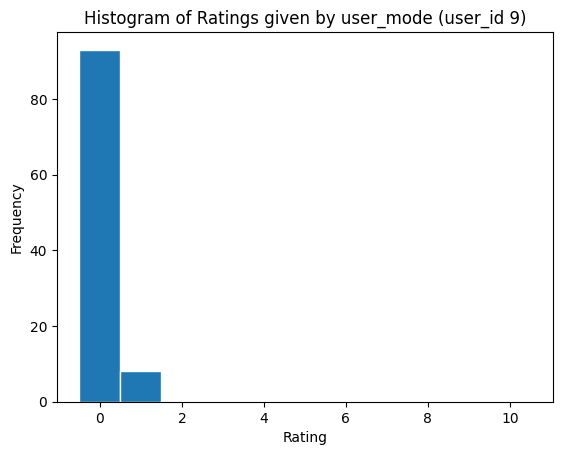In [ ]:
# Histogram of ratings of item_mode
plt.hist(train_rating[index_item[item_mode]], edgecolor='white', align='left',
bins=np.arange(start=rating_lower_bound, stop=rating_upper_bound+2, step=1))
plt.xlabel("Rating")
plt.ylabel("Frequency")
plt.title('Histogram of Ratings given by item_mode (item_id 786)')
plt.savefig('EDA_plot_2.png')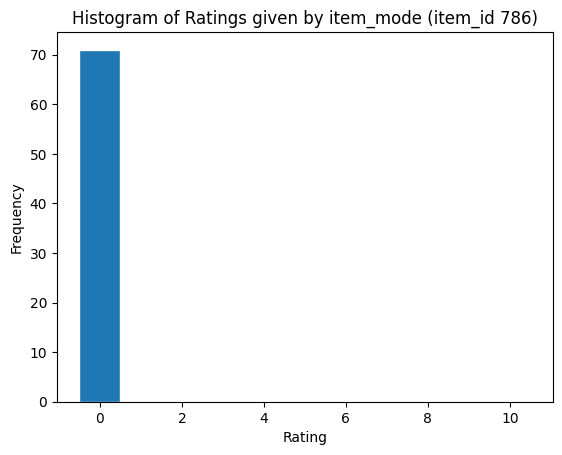In [ ]:
# Check the variance of ratings given by each user in record
var_user = [np.var(train_rating[index_user[u]]) for u in set(train_pair[:,0])]
plt.hist(var_user, edgecolor='white')
plt.xlabel("Variance")
plt.ylabel("Count")
plt.title('Variance of ratings given by users in train data')
plt.savefig('EDA_plot_3.png')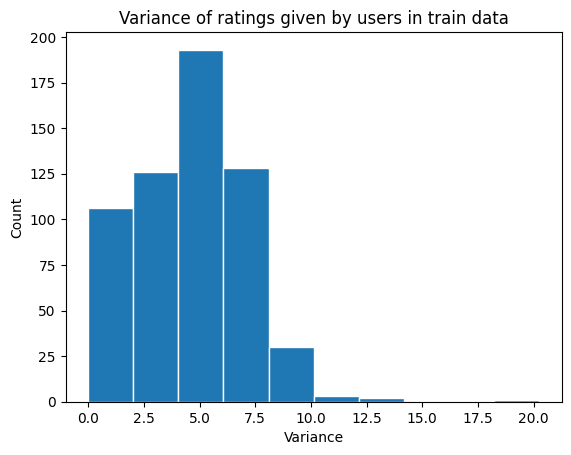In [ ]:
# Check the variance of ratings of each item in record
var_item = [np.var(train_rating[index_item[i]]) for i in set(train_pair[:,1])]
plt.hist(var_item, edgecolor='white')
plt.xlabel("Variance")
plt.ylabel("Count")
plt.title('Variance of ratings of items in train data')
plt.savefig('EDA_plot_4.png')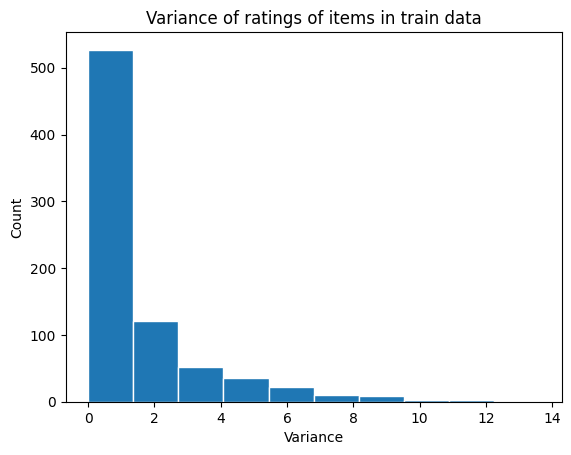In [ ]:
# Distribution of ratings
plt.hist(train_rating,edgecolor='white', align='left',
bins=np.arange(start=rating_lower_bound, stop=rating_upper_bound+2, step=1))
plt.xlabel("Rating")
plt.ylabel("Frequency")
plt.title('Distribution of Ratings')
plt.savefig('EDA_plot_5.png')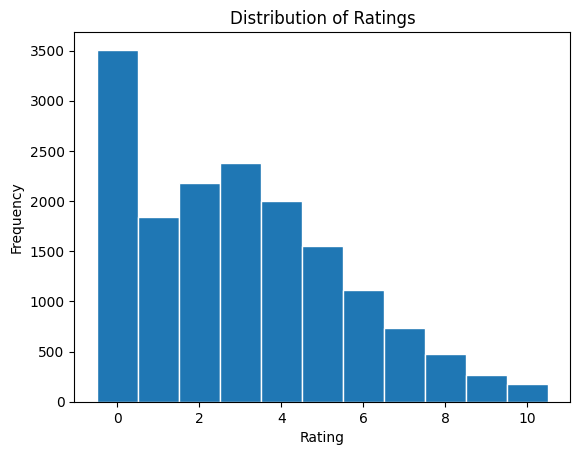In [ ]:
# Item popularity
# Item mean against number of ratings received by item
item_set = list(set(train_pair[:,1]))
n_item_train = len(item_set)
aver_ratings = np.ones(n_item_train)
n_ratings = np.ones(n_item_train)
for i in range(n_item_train):
item_index_temp = index_item[item_set[i]]
aver_ratings[i]=np.mean(train_rating[item_index_temp])
n_ratings[i]=len(item_index_temp)

plt.scatter(n_ratings, aver_ratings)
plt.xlabel("Number of ratings")
plt.ylabel("Average rating")
plt.title('Average rating against item popularity')
plt.savefig('EDA_plot_6.png')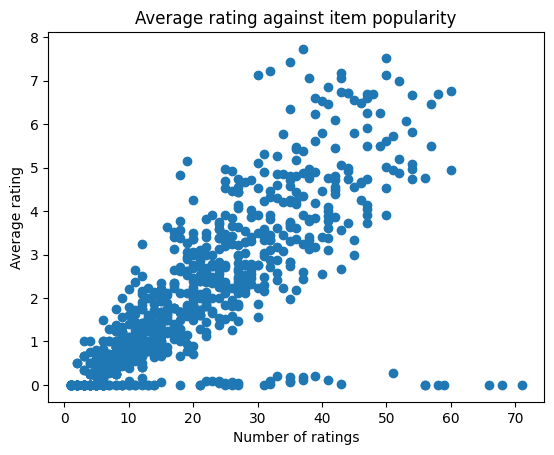In [ ]:
# User activeness
# User mean against number of ratings given by user
user_set = list(set(train_pair[:,0]))
n_user_train = len(user_set)
aver_ratings = np.ones(n_item_train)
n_ratings = np.ones(n_item_train)
for u in range(n_user_train):
user_index_temp = index_user[user_set[u]]
aver_ratings[u] = np.mean(train_rating[user_index_temp])
n_ratings[u] = len(user_index_temp)

plt.scatter(n_ratings, aver_ratings)
plt.xlabel("Number of ratings")
plt.ylabel("Average rating")
plt.title('Average rating against user activeness')
plt.savefig('EDA_plot_7.png')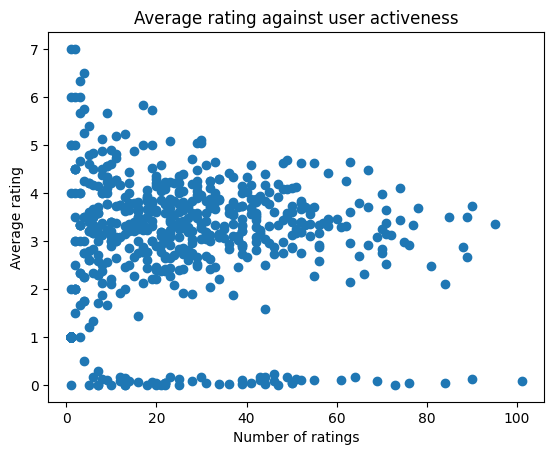## Appendix B¶

Codes for Model 1

##### Appendix B1¶

Codes for ALS in lecture - generate a set of parameters (for reference only) to get a sense of the parameter grid for Model 1.1

In [ ]:
class user_item_MF(object):
def __init__(self, n_user, n_item, lam=.001, K=10, iterNum=50, tol=1e-4, verbose=1, user_mean=True, item_mean=True):
self.P = np.random.randn(n_user, K)
self.Q = np.random.randn(n_item, K)
self.n_user = n_user
self.n_item = n_item
self.user_mean=user_mean
self.item_mean=item_mean
if(user_mean):
self.mu=np.random.randn(n_user)
else:
self.mu=np.zeros(n_user)
if(item_mean):
self.rho=np.random.randn(n_item)
else:
self.rho=np.zeros(n_item)
self.lam = lam
self.K = K
self.iterNum = iterNum
self.tol = tol
self.verbose = verbose

def fit(self,train_pair,train_rating):
diff, tol=0, self.tol
iterNum=self.iterNum
n_item=self.n_item
n_user=self.n_user
K=self.K
lam=self.lam

index_item = [np.where(train_pair[:,1] == i) for i in range(n_item)]
index_user = [np.where(train_pair[:,0] == u) for u in range(n_user)]

if(self.verbose==1):
print('Fitting User-Item-MF: K: %d, lam: %.5f' %(K, lam))

for iter in range(iterNum):
obj_old=self.obj(test_pair=train_pair,test_rating=train_rating)
#udpate mu
if(self.user_mean):
for u in range(n_user):
ind_user_temp=index_user[u]
if(len(ind_user_temp)==0):
self.mu[u]=0
continue
temp= train_rating[ind_user_temp] - self.rho[train_pair[ind_user_temp,1]] - np.dot(self.Q[train_pair[ind_user_temp,1],:],self.P[u])
self.mu[u]=np.mean(temp)

#update rho
if(self.item_mean):
for i in range(n_item):
ind_item_temp=index_item[i]
if(len(ind_item_temp)==0):
self.rho[i]=0
continue
temp= train_rating[ind_item_temp] - self.mu[train_pair[ind_item_temp,0]] - np.dot(self.P[train_pair[ind_item_temp,0],:],self.Q[i])
self.rho[i]=np.mean(temp)

#update P
for u in range(n_user):
ind_user_temp=index_user[u]
sum_matrix_qi=np.zeros((K,K))
sum_qi=np.zeros(K)
if(len(ind_user_temp)==0):
self.P[u,:]=np.zeros(K)
continue
for record in ind_user_temp:
item_temp=train_pair[record,1]
rating_temp=train_rating[record]
sum_matrix_qi=sum_matrix_qi+np.outer(self.Q[item_temp,:],self.Q[item_temp,:])
sum_qi=sum_qi+(rating_temp-self.mu[u]-self.rho[item_temp])*self.Q[item_temp,:]
self.P[u,:]=np.linalg.inv( sum_matrix_qi + lam*len(train_rating)*np.identity(K)) @ sum_qi

#update Q
for i in range(n_item):
ind_item_temp=index_item[i]
sum_matrix_pu=np.zeros((K,K))
sum_pu=np.zeros(K)
if(len(ind_item_temp)==0):
self.Q[i,:]=np.zeros(K)
continue
for record in ind_item_temp:
user_temp=train_pair[record,0]
rating_temp=train_rating[record]
sum_matrix_pu=sum_matrix_pu+np.outer(self.P[user_temp,:],self.P[user_temp,:])
sum_pu=sum_pu+(rating_temp-self.mu[user_temp]-self.rho[i])*self.P[user_temp,:]
self.Q[i,:]=np.linalg.inv( sum_matrix_pu + lam*len(train_rating)*np.identity(K)) @ sum_pu

obj_new=self.obj(test_pair=train_pair,test_rating=train_rating)
diff=abs(obj_old-obj_new)
if self.verbose:
print("User-Item-MF: ite: %d; diff: %.3f Obj: %.3f" %(iter, diff, obj_new))
if(diff < tol):
break

def obj(self, test_pair, test_rating):
return self.rmse(test_pair=test_pair, test_rating=test_rating)**2 + self.lam*( np.sum((self.P)**2) + np.sum((self.Q)**2))

def rmse(self, test_pair, test_rating):
pred=self.predict(test_pair=test_pair)
return(np.sqrt(np.mean((test_rating-pred)**2)))

def predict(self,test_pair):
pred_rating=[self.mu[record]+self.rho[record]+np.dot(self.P[record,:],self.Q[record,:]) for record in test_pair]
return(np.array(pred_rating))

In [ ]:
from sklearn.model_selection import KFold
import itertools
import pandas as pd
import seaborn as sns
import matplotlib.pyplot as plt

class MF_CV(object):

def __init__(self, n_user, n_item, cv=5,
lams=[.000001,.0001,.001,.01], # lams and Ks are vectors (our grid)
Ks=[3,5,10,20],
iterNum=10, tol=1e-4):
# self.index_item = []
# self.index_user = []
self.n_user = n_user
self.n_item = n_item
self.cv = cv
self.lams = lams
self.Ks = Ks
self.iterNum = iterNum
self.tol = tol
self.best_model = {}
self.cv_result = {'K': [], 'lam': [], 'train_rmse': [], 'valid_rmse': []} # our result

def grid_search(self, train_pair, train_rating):
## generate all comb of K and lam
kf = KFold(n_splits=self.cv, shuffle=True)
for (K,lam) in itertools.product(self.Ks, self.lams):
train_rmse_tmp, valid_rmse_tmp = 0., 0.
for train_index, valid_index in kf.split(train_pair):
# produce training/validation sets
train_pair_cv, train_rating_cv = train_pair[train_index], train_rating[train_index]
valid_pair_cv, valid_rating_cv = train_pair[valid_index], train_rating[valid_index]
# fit the model based on CV data
model_tmp = user_item_MF(self.n_user, self.n_item, K=K, lam=lam, verbose=0)
model_tmp.fit(train_pair=train_pair_cv, train_rating=train_rating_cv)
## valid model in the validation set
train_rmse_tmp_cv = model_tmp.rmse(train_pair_cv, train_rating_cv)
valid_rmse_tmp_cv = model_tmp.rmse(valid_pair_cv, valid_rating_cv)
train_rmse_tmp += train_rmse_tmp_cv/self.cv
valid_rmse_tmp += valid_rmse_tmp_cv/self.cv
print('%d-Fold CV for K: %d; lam: %.5f: train_rmse: %.3f, valid_rmse: %.3f'
%(self.cv, K, lam, train_rmse_tmp_cv, valid_rmse_tmp_cv))
self.cv_result['K'].append(K)
self.cv_result['lam'].append(lam)
self.cv_result['train_rmse'].append(train_rmse_tmp)
self.cv_result['valid_rmse'].append(valid_rmse_tmp)
self.cv_result = pd.DataFrame.from_dict(self.cv_result)
best_ind = self.cv_result['valid_rmse'].argmin()
self.best_model = self.cv_result.loc[best_ind]

def plot_grid(self, data_source='valid'):
sns.set_theme()
if data_source == 'train':
cv_pivot = self.cv_result.pivot("K", "lam", "train_rmse")
elif data_source == 'valid':
cv_pivot = self.cv_result.pivot("K", "lam", "valid_rmse")
else:
raise ValueError('data_source must be train or valid!')
sns.heatmap(cv_pivot, annot=True, fmt=".3f", linewidths=.5, cmap="YlGnBu")
plt.show()

In [ ]:
Ks, lams = [3, 5, 10], 10**np.arange(-5, -1, .5)

MF_cv = MF_CV(n_user, n_item, cv=5, Ks=Ks, lams=lams)
MF_cv.grid_search(train_pair, train_rating)

5-Fold CV for K: 3; lam: 0.00001: train_rmse: 0.416, valid_rmse: 0.892
5-Fold CV for K: 3; lam: 0.00001: train_rmse: 0.411, valid_rmse: 0.959
5-Fold CV for K: 3; lam: 0.00001: train_rmse: 0.418, valid_rmse: 0.906
5-Fold CV for K: 3; lam: 0.00001: train_rmse: 0.414, valid_rmse: 0.991
5-Fold CV for K: 3; lam: 0.00001: train_rmse: 0.414, valid_rmse: 0.936
5-Fold CV for K: 3; lam: 0.00003: train_rmse: 0.417, valid_rmse: 0.864
5-Fold CV for K: 3; lam: 0.00003: train_rmse: 0.420, valid_rmse: 0.842
5-Fold CV for K: 3; lam: 0.00003: train_rmse: 0.423, valid_rmse: 0.822
5-Fold CV for K: 3; lam: 0.00003: train_rmse: 0.422, valid_rmse: 0.826
5-Fold CV for K: 3; lam: 0.00003: train_rmse: 0.420, valid_rmse: 0.817
5-Fold CV for K: 3; lam: 0.00010: train_rmse: 0.439, valid_rmse: 0.796
5-Fold CV for K: 3; lam: 0.00010: train_rmse: 0.445, valid_rmse: 0.777
5-Fold CV for K: 3; lam: 0.00010: train_rmse: 0.440, valid_rmse: 0.763
5-Fold CV for K: 3; lam: 0.00010: train_rmse: 0.439, valid_rmse: 0.774
5-Fold CV for K: 3; lam: 0.00010: train_rmse: 0.437, valid_rmse: 0.745
5-Fold CV for K: 3; lam: 0.00032: train_rmse: 0.526, valid_rmse: 0.735
5-Fold CV for K: 3; lam: 0.00032: train_rmse: 0.525, valid_rmse: 0.752
5-Fold CV for K: 3; lam: 0.00032: train_rmse: 0.523, valid_rmse: 0.754
5-Fold CV for K: 3; lam: 0.00032: train_rmse: 0.521, valid_rmse: 0.769
5-Fold CV for K: 3; lam: 0.00032: train_rmse: 0.525, valid_rmse: 0.747
5-Fold CV for K: 3; lam: 0.00100: train_rmse: 0.727, valid_rmse: 0.877
5-Fold CV for K: 3; lam: 0.00100: train_rmse: 0.723, valid_rmse: 0.902
5-Fold CV for K: 3; lam: 0.00100: train_rmse: 0.725, valid_rmse: 0.884
5-Fold CV for K: 3; lam: 0.00100: train_rmse: 0.722, valid_rmse: 0.866
5-Fold CV for K: 3; lam: 0.00100: train_rmse: 0.727, valid_rmse: 0.860
5-Fold CV for K: 3; lam: 0.00316: train_rmse: 0.923, valid_rmse: 1.035
5-Fold CV for K: 3; lam: 0.00316: train_rmse: 0.925, valid_rmse: 1.038
5-Fold CV for K: 3; lam: 0.00316: train_rmse: 0.927, valid_rmse: 1.027
5-Fold CV for K: 3; lam: 0.00316: train_rmse: 0.916, valid_rmse: 1.067
5-Fold CV for K: 3; lam: 0.00316: train_rmse: 0.919, valid_rmse: 1.065
5-Fold CV for K: 3; lam: 0.01000: train_rmse: 0.919, valid_rmse: 1.056
5-Fold CV for K: 3; lam: 0.01000: train_rmse: 0.916, valid_rmse: 1.073
5-Fold CV for K: 3; lam: 0.01000: train_rmse: 0.927, valid_rmse: 1.032
5-Fold CV for K: 3; lam: 0.01000: train_rmse: 0.929, valid_rmse: 1.018
5-Fold CV for K: 3; lam: 0.01000: train_rmse: 0.921, valid_rmse: 1.038
5-Fold CV for K: 3; lam: 0.03162: train_rmse: 0.925, valid_rmse: 1.032
5-Fold CV for K: 3; lam: 0.03162: train_rmse: 0.922, valid_rmse: 1.050
5-Fold CV for K: 3; lam: 0.03162: train_rmse: 0.923, valid_rmse: 1.034
5-Fold CV for K: 3; lam: 0.03162: train_rmse: 0.917, valid_rmse: 1.072
5-Fold CV for K: 3; lam: 0.03162: train_rmse: 0.925, valid_rmse: 1.025
5-Fold CV for K: 5; lam: 0.00001: train_rmse: 0.301, valid_rmse: 1.062
5-Fold CV for K: 5; lam: 0.00001: train_rmse: 0.307, valid_rmse: 1.096
5-Fold CV for K: 5; lam: 0.00001: train_rmse: 0.303, valid_rmse: 1.077
5-Fold CV for K: 5; lam: 0.00001: train_rmse: 0.304, valid_rmse: 1.036
5-Fold CV for K: 5; lam: 0.00001: train_rmse: 0.300, valid_rmse: 1.055
5-Fold CV for K: 5; lam: 0.00003: train_rmse: 0.313, valid_rmse: 0.903
5-Fold CV for K: 5; lam: 0.00003: train_rmse: 0.319, valid_rmse: 0.909
5-Fold CV for K: 5; lam: 0.00003: train_rmse: 0.308, valid_rmse: 0.952
5-Fold CV for K: 5; lam: 0.00003: train_rmse: 0.315, valid_rmse: 0.901
5-Fold CV for K: 5; lam: 0.00003: train_rmse: 0.310, valid_rmse: 0.911
5-Fold CV for K: 5; lam: 0.00010: train_rmse: 0.354, valid_rmse: 0.802
5-Fold CV for K: 5; lam: 0.00010: train_rmse: 0.354, valid_rmse: 0.810
5-Fold CV for K: 5; lam: 0.00010: train_rmse: 0.355, valid_rmse: 0.784
5-Fold CV for K: 5; lam: 0.00010: train_rmse: 0.349, valid_rmse: 0.818
5-Fold CV for K: 5; lam: 0.00010: train_rmse: 0.353, valid_rmse: 0.783
5-Fold CV for K: 5; lam: 0.00032: train_rmse: 0.484, valid_rmse: 0.745
5-Fold CV for K: 5; lam: 0.00032: train_rmse: 0.488, valid_rmse: 0.759
5-Fold CV for K: 5; lam: 0.00032: train_rmse: 0.486, valid_rmse: 0.765
5-Fold CV for K: 5; lam: 0.00032: train_rmse: 0.486, valid_rmse: 0.785
5-Fold CV for K: 5; lam: 0.00032: train_rmse: 0.485, valid_rmse: 0.766
5-Fold CV for K: 5; lam: 0.00100: train_rmse: 0.726, valid_rmse: 0.879
5-Fold CV for K: 5; lam: 0.00100: train_rmse: 0.723, valid_rmse: 0.859
5-Fold CV for K: 5; lam: 0.00100: train_rmse: 0.727, valid_rmse: 0.883
5-Fold CV for K: 5; lam: 0.00100: train_rmse: 0.726, valid_rmse: 0.884
5-Fold CV for K: 5; lam: 0.00100: train_rmse: 0.723, valid_rmse: 0.905
5-Fold CV for K: 5; lam: 0.00316: train_rmse: 0.917, valid_rmse: 1.062
5-Fold CV for K: 5; lam: 0.00316: train_rmse: 0.926, valid_rmse: 1.044
5-Fold CV for K: 5; lam: 0.00316: train_rmse: 0.918, valid_rmse: 1.070
5-Fold CV for K: 5; lam: 0.00316: train_rmse: 0.925, valid_rmse: 1.034
5-Fold CV for K: 5; lam: 0.00316: train_rmse: 0.925, valid_rmse: 1.032
5-Fold CV for K: 5; lam: 0.01000: train_rmse: 0.923, valid_rmse: 1.040
5-Fold CV for K: 5; lam: 0.01000: train_rmse: 0.925, valid_rmse: 1.026
5-Fold CV for K: 5; lam: 0.01000: train_rmse: 0.917, valid_rmse: 1.064
5-Fold CV for K: 5; lam: 0.01000: train_rmse: 0.926, valid_rmse: 1.026
5-Fold CV for K: 5; lam: 0.01000: train_rmse: 0.922, valid_rmse: 1.052
5-Fold CV for K: 5; lam: 0.03162: train_rmse: 0.919, valid_rmse: 1.059
5-Fold CV for K: 5; lam: 0.03162: train_rmse: 0.926, valid_rmse: 1.024
5-Fold CV for K: 5; lam: 0.03162: train_rmse: 0.927, valid_rmse: 1.045
5-Fold CV for K: 5; lam: 0.03162: train_rmse: 0.917, valid_rmse: 1.074
5-Fold CV for K: 5; lam: 0.03162: train_rmse: 0.923, valid_rmse: 1.041
5-Fold CV for K: 10; lam: 0.00001: train_rmse: 0.109, valid_rmse: 1.134
5-Fold CV for K: 10; lam: 0.00001: train_rmse: 0.102, valid_rmse: 1.163
5-Fold CV for K: 10; lam: 0.00001: train_rmse: 0.108, valid_rmse: 1.166
5-Fold CV for K: 10; lam: 0.00001: train_rmse: 0.114, valid_rmse: 1.230
5-Fold CV for K: 10; lam: 0.00001: train_rmse: 0.104, valid_rmse: 1.172
5-Fold CV for K: 10; lam: 0.00003: train_rmse: 0.132, valid_rmse: 0.950
5-Fold CV for K: 10; lam: 0.00003: train_rmse: 0.133, valid_rmse: 0.947
5-Fold CV for K: 10; lam: 0.00003: train_rmse: 0.131, valid_rmse: 0.950
5-Fold CV for K: 10; lam: 0.00003: train_rmse: 0.132, valid_rmse: 0.925
5-Fold CV for K: 10; lam: 0.00003: train_rmse: 0.132, valid_rmse: 0.924
5-Fold CV for K: 10; lam: 0.00010: train_rmse: 0.228, valid_rmse: 0.816
5-Fold CV for K: 10; lam: 0.00010: train_rmse: 0.229, valid_rmse: 0.781
5-Fold CV for K: 10; lam: 0.00010: train_rmse: 0.226, valid_rmse: 0.806
5-Fold CV for K: 10; lam: 0.00010: train_rmse: 0.227, valid_rmse: 0.808
5-Fold CV for K: 10; lam: 0.00010: train_rmse: 0.231, valid_rmse: 0.789
5-Fold CV for K: 10; lam: 0.00032: train_rmse: 0.447, valid_rmse: 0.761
5-Fold CV for K: 10; lam: 0.00032: train_rmse: 0.447, valid_rmse: 0.746
5-Fold CV for K: 10; lam: 0.00032: train_rmse: 0.445, valid_rmse: 0.787
5-Fold CV for K: 10; lam: 0.00032: train_rmse: 0.446, valid_rmse: 0.789
5-Fold CV for K: 10; lam: 0.00032: train_rmse: 0.446, valid_rmse: 0.765
5-Fold CV for K: 10; lam: 0.00100: train_rmse: 0.727, valid_rmse: 0.887
5-Fold CV for K: 10; lam: 0.00100: train_rmse: 0.727, valid_rmse: 0.872
5-Fold CV for K: 10; lam: 0.00100: train_rmse: 0.724, valid_rmse: 0.882
5-Fold CV for K: 10; lam: 0.00100: train_rmse: 0.722, valid_rmse: 0.866
5-Fold CV for K: 10; lam: 0.00100: train_rmse: 0.725, valid_rmse: 0.884
5-Fold CV for K: 10; lam: 0.00316: train_rmse: 0.927, valid_rmse: 1.027
5-Fold CV for K: 10; lam: 0.00316: train_rmse: 0.919, valid_rmse: 1.051
5-Fold CV for K: 10; lam: 0.00316: train_rmse: 0.921, valid_rmse: 1.045
5-Fold CV for K: 10; lam: 0.00316: train_rmse: 0.922, valid_rmse: 1.043
5-Fold CV for K: 10; lam: 0.00316: train_rmse: 0.923, valid_rmse: 1.047
5-Fold CV for K: 10; lam: 0.01000: train_rmse: 0.929, valid_rmse: 1.015
5-Fold CV for K: 10; lam: 0.01000: train_rmse: 0.922, valid_rmse: 1.046
5-Fold CV for K: 10; lam: 0.01000: train_rmse: 0.924, valid_rmse: 1.027
5-Fold CV for K: 10; lam: 0.01000: train_rmse: 0.918, valid_rmse: 1.062
5-Fold CV for K: 10; lam: 0.01000: train_rmse: 0.919, valid_rmse: 1.051
5-Fold CV for K: 10; lam: 0.03162: train_rmse: 0.927, valid_rmse: 1.033
5-Fold CV for K: 10; lam: 0.03162: train_rmse: 0.926, valid_rmse: 1.042
5-Fold CV for K: 10; lam: 0.03162: train_rmse: 0.921, valid_rmse: 1.052
5-Fold CV for K: 10; lam: 0.03162: train_rmse: 0.916, valid_rmse: 1.058
5-Fold CV for K: 10; lam: 0.03162: train_rmse: 0.921, valid_rmse: 1.054

In [ ]:
MF_cv.plot_grid()

<ipython-input-42-5f6b383dc78a>:57: FutureWarning: In a future version of pandas all arguments of DataFrame.pivot will be keyword-only.
cv_pivot = self.cv_result.pivot("K", "lam", "valid_rmse")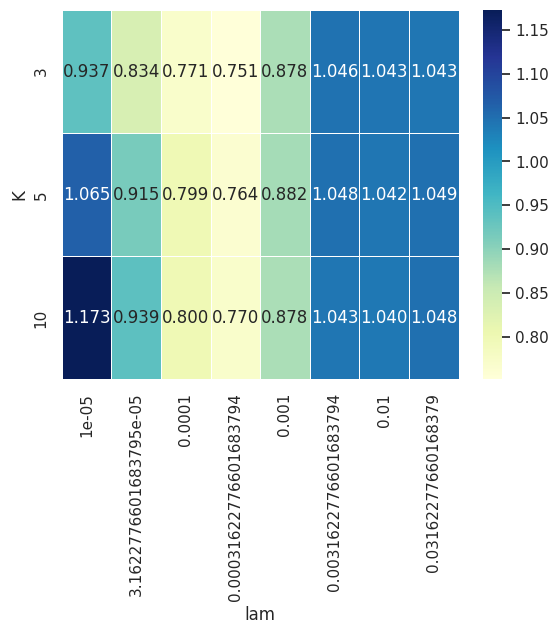Insight: both K and λ are small.

##### Appendix B2¶

Codes for Model 1.1

Surprise and auto-surprise libraries are used from now on.

In [ ]:
# Import libraries
from surprise.model_selection import GridSearchCV
from surprise.dataset import DatasetAutoFolds
from surprise import Dataset
from surprise import SVD

trainset = DatasetAutoFolds.build_full_trainset(data)

In [ ]:
# GridSearch Begins with the following parameter gird
param_grid = {"n_factors": [3, 5, 10, 40, 70, 100],
"biased": ['True'],
"n_epochs": ,
"lr_all": [0.0001, 0.001, 0.005, 0.01, 0.1],
"reg_all": [0.0001, 0.001, 0.1, 0.5, 1, 2],
"reg_bu": ,
"reg_bi": 
}

gs = GridSearchCV(SVD, param_grid, cv=5,n_jobs =-1, joblib_verbose =2)
gs.fit(data)
print(gs.best_score["rmse"])
print(gs.best_params["rmse"])

[Parallel(n_jobs=-1)]: Using backend LokyBackend with 2 concurrent workers.
[Parallel(n_jobs=-1)]: Done  37 tasks      | elapsed:   20.5s
[Parallel(n_jobs=-1)]: Done 158 tasks      | elapsed:  1.3min
[Parallel(n_jobs=-1)]: Done 361 tasks      | elapsed:  3.2min
[Parallel(n_jobs=-1)]: Done 644 tasks      | elapsed:  7.6min

0.6951833481595394
{'n_factors': 3, 'biased': 'True', 'n_epochs': 200, 'lr_all': 0.005, 'reg_all': 0.1, 'reg_bu': 0, 'reg_bi': 0}
CPU times: user 22.6 s, sys: 1.78 s, total: 24.4 s
Wall time: 13min 57s

[Parallel(n_jobs=-1)]: Done 900 out of 900 | elapsed: 13.9min finished

In [ ]:
param_grid = {"n_factors": , 'biased': ['True'],"n_epochs": ,
"lr_all": [0.003, 0.005, 0.007],
"reg_all": [0.01, 0.1, 0.3],
"reg_bu": , "reg_bi": 
}

gs = GridSearchCV(SVD, param_grid, cv=5,n_jobs =-1, joblib_verbose =2)
gs.fit(data)
print(gs.best_score["rmse"])
print(gs.best_params["rmse"])

[Parallel(n_jobs=-1)]: Using backend LokyBackend with 2 concurrent workers.
[Parallel(n_jobs=-1)]: Done  37 tasks      | elapsed:   22.4s

0.6859022409948813
{'n_factors': 3, 'biased': 'True', 'n_epochs': 200, 'lr_all': 0.005, 'reg_all': 0.1, 'reg_bu': 0, 'reg_bi': 0}

[Parallel(n_jobs=-1)]: Done  45 out of  45 | elapsed:   25.3s finished

In [ ]:
param_grid = {"n_factors": , 'biased': ['True'],"n_epochs": ,
"lr_all": [0.004, 0.005, 0.006],
"reg_all": [0.08, 0.1, 0.15],
"reg_bu": , "reg_bi": 
}

gs = GridSearchCV(SVD, param_grid, cv=5,n_jobs =-1, joblib_verbose =2)
gs.fit(data)
print(gs.best_score["rmse"])
print(gs.best_params["rmse"])

[Parallel(n_jobs=-1)]: Using backend LokyBackend with 2 concurrent workers.
[Parallel(n_jobs=-1)]: Done  37 tasks      | elapsed:   19.0s

0.6866348712491878
{'n_factors': 3, 'biased': 'True', 'n_epochs': 200, 'lr_all': 0.005, 'reg_all': 0.15, 'reg_bu': 0, 'reg_bi': 0}

[Parallel(n_jobs=-1)]: Done  45 out of  45 | elapsed:   22.2s finished

In [ ]:
param_grid = {"n_factors": , 'biased': ['True'],"n_epochs": ,
"lr_all": [0.005], "reg_all": [0.14, 0.15,0.16],
"reg_bu": , "reg_bi": 
}

gs = GridSearchCV(SVD, param_grid, cv=5,n_jobs =-1, joblib_verbose =2)
gs.fit(data)
print(gs.best_score["rmse"])
print(gs.best_params["rmse"])

[Parallel(n_jobs=-1)]: Using backend LokyBackend with 2 concurrent workers.

0.6905183951815582
{'n_factors': 3, 'biased': 'True', 'n_epochs': 200, 'lr_all': 0.005, 'reg_all': 0.14, 'reg_bu': 0, 'reg_bi': 0}

[Parallel(n_jobs=-1)]: Done  15 out of  15 | elapsed:    9.4s finished

In [ ]:
param_grid = {"n_factors": , 'biased': ['True'],
"n_epochs": [200,600,900,1200,1400,2000],
"lr_all": [0.005], "reg_all": [0.1,0.14, 0.15],
"reg_bu": , "reg_bi": 
}

gs = GridSearchCV(SVD, param_grid, cv=5,n_jobs =-1, joblib_verbose =2)
gs.fit(data)
print(gs.best_score["rmse"])
print(gs.best_params["rmse"])

[Parallel(n_jobs=-1)]: Using backend LokyBackend with 2 concurrent workers.
[Parallel(n_jobs=-1)]: Done  37 tasks      | elapsed:   46.5s

0.6838662190742203
{'n_factors': 3, 'biased': 'True', 'n_epochs': 200, 'lr_all': 0.005, 'reg_all': 0.1, 'reg_bu': 0, 'reg_bi': 0}

[Parallel(n_jobs=-1)]: Done  90 out of  90 | elapsed:  3.7min finished

In [ ]:
param_grid = {"n_factors": [3,10,50], 'biased': ['True'],
"n_epochs": [200,500,1300,2000],
"lr_all": [0.005], "reg_all": [0.1],
"reg_bu": , "reg_bi": 
}

gs = GridSearchCV(SVD, param_grid, cv=5,n_jobs =-1, joblib_verbose =2)
gs.fit(data)
print(gs.best_score["rmse"])
print(gs.best_params["rmse"])

[Parallel(n_jobs=-1)]: Using backend LokyBackend with 2 concurrent workers.
[Parallel(n_jobs=-1)]: Done  37 tasks      | elapsed:  1.5min

0.6887168139244468
{'n_factors': 50, 'biased': 'True', 'n_epochs': 2000, 'lr_all': 0.005, 'reg_all': 0.1, 'reg_bu': 0, 'reg_bi': 0}

[Parallel(n_jobs=-1)]: Done  60 out of  60 | elapsed:  3.7min finished

In [ ]:
param_grid = {"n_factors": , 'biased': ['True'],
"n_epochs": [200,500,1300,2000], "lr_all": [0.005],
"reg_all": [0.1], "reg_bu": , "reg_bi": 
}

gs = GridSearchCV(SVD, param_grid, cv=5,n_jobs =-1, joblib_verbose =2)
gs.fit(data)
print(gs.best_score["rmse"])
print(gs.best_params["rmse"])

[Parallel(n_jobs=-1)]: Using backend LokyBackend with 2 concurrent workers.

0.6935234346734008
{'n_factors': 10, 'biased': 'True', 'n_epochs': 1300, 'lr_all': 0.005, 'reg_all': 0.1, 'reg_bu': 0, 'reg_bi': 0}

[Parallel(n_jobs=-1)]: Done  20 out of  20 | elapsed:  1.0min finished

In [ ]:
# Apply the finalize parameter set generated by GridSearch
svd = SVD(n_factors=3, biased=True, n_epochs=200, lr_all=0.005, reg_all=0.1, reg_bu=0, reg_bi=0)
svd.fit(trainset)

# Predict the ratings
predicted_rating = np.zeros(len(test_pair[:,1]))
for i in range(len(test_pair[:,1])):
predicted_rating[i] = svd.predict(test_pair[i,0],test_pair[i,1]).est

# Output the results
sub['rating'] = predicted_rating
sub.to_csv('sub3.csv', index=False)

##### Appendix B3¶

Codes for Model 1.2

In [ ]:
# Import library
from auto_surprise.engine import Engine
import hyperopt

In [ ]:
# Intitialize auto surprise engine
engine = Engine(verbose=True, algorithms=['svd'])

# Start the trainer
# Set maximum evaluations be 100
# TPE  algorithm is applied
best_algo, best_params, best_score, tasks = engine.train(
data=data,
target_metric='test_rmse',
cpu_time_limit=60*60*2,
max_evals=100,
hpo_algo=hyperopt.tpe.suggest
)